Next: Information theoretic approach Up: Bayesian methods for data Previous: EM algorithm   Contents

# Ensemble learning

Ensemble learning is a relatively new concept suggested by Hinton and van Camp in 1993 . It allows approximating the true posterior distribution with a tractable approximation and fitting it to the actual probability mass with no intermediate point estimates.

The posterior distribution of the model parameters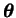,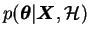, is approximated with another distribution or approximating ensemble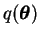. The objective function chosen to measure the quality of the approximation is essentially the same cost function as the one for EM algorithm in Equation (3.10)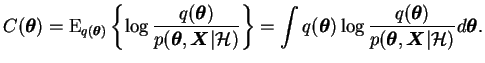(3.11)

Ensemble learning is based on finding an optimal function to approximate another function. Such optimisation methods are called variational methods and therefore ensemble learning is sometimes also called variational learning .

A closer look at the cost function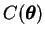shows that it can be represented as a sum of two simple terms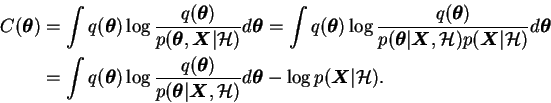(3.12)

The first term in Equation (3.12) is the Kullback-Leibler divergence between the approximate posteriorand the true posterior. A simple application of Jensen's inequality  shows that the Kullback-Leibler divergence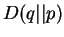between two distributionsand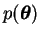is always nonnegative: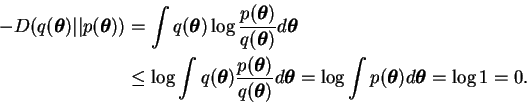(3.13)

Since the logarithm is a strictly concave function, the equality holds if and only if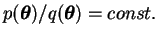, i.e.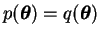.

The Kullback-Leibler divergence is not symmetric and it does not obey the triangle inequality, so it is not a metric. Nevertheless it can be considered a kind of a distance measure between probability distributions .

Using the inequality in Equation (3.13) we find that the cost functionis bounded from below by the negative logarithm of the evidence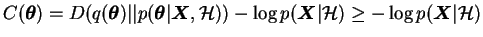(3.14)

and there is equality if and only if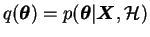.

Looking at this the other way round, the cost function gives a lower bound on the model evidence with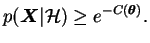(3.15)

The error of this estimate is governed by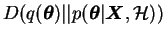. Assuming the distributionhas been optimised to fit well to the true posterior, the error should be rather small. Therefore it is possible to approximate the evidence by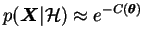. This allows using the values of the cost function for model selection as presented in Section 3.2.1 .

An important feature for practical use of ensemble learning is that the cost function and its derivatives with respect to the parameters of the approximating distribution can be easily evaluated for many models. Hinton and van Camp  used a separable Gaussian approximating distribution for a single hidden layer MLP network. After that many authors have used the method for different applications.

SubsectionsNext: Information theoretic approach Up: Bayesian methods for data Previous: EM algorithm   Contents
Antti Honkela 2001-05-30